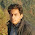## Wednesday, January 25, 2012

This mini project is written in C++ where you find mainly two classes one class item another class amount and class amount is inheritance form class item.It is simple console application without graphics. From this project you learn file handling in c++ and use of stream class.And main defect of this program is that goto label  is used to jump form one menu to another menu and separate function for editing  and deleting items are not used.So,if you want to make it your school project or college mini project then modify it ,make separate function for editing and deleting and also try to use while loop instead of goto label .Any suggestion and help for this project is appreciated .
Here is the source code ,copy and compile it in gcc with code::blocks IDE or Download project from GitHub.

`//program for creating bill in a supermarket`
`#include<iostream>`
`#include<windows.h>`
`#include<conio.h>`
`#include<fstream>`
`#include<cstring>`
`#include<cstdio>`
`#include<cstdlib>`
`#include<iomanip>`
`using namespace std;`
`//global variable declaration`
`int k=7,r=0,flag=0;`
`COORD coord = {0, 0};`
` `
`void gotoxy(int x, int y)`
`{`
` COORD coord;`
` coord.X = x;`
` coord.Y = y;`
` SetConsoleCursorPosition(GetStdHandle(STD_OUTPUT_HANDLE), coord);`
`}`
`struct date`
`{int mm,dd,yy;};`
` `
`ofstream fout;`
`ifstream fin;`
` `
`class item`
`{`
`int itemno;`
`char name;`
`date d;`
`public:`
`void add()`
`{`
`cout<<"\n\n\tItem No: ";`
`cin>>itemno;`
`cout<<"\n\n\tName of the item: ";`
`cin>>name;`
`//gets(name);`
`cout<<"\n\n\tManufacturing Date(dd-mm-yy): ";`
`cin>>d.mm>>d.dd>>d.yy;`
`}`
`void show()`
`{`
`cout<<"\n\tItem No: ";`
`cout<<itemno;`
`cout<<"\n\n\tName of the item: ";`
`cout<<name;`
`cout<<"\n\n\tDate : ";`
`cout<<d.mm<<"-"<<d.dd<<"-"<<d.yy;`
`}`
`void report()`
`{`
`gotoxy(3,k);`
`cout<<itemno;`
`gotoxy(13,k);`
`puts(name);`
`}`
`int retno()`
`{`
`    return(itemno);`
` `
`}`
` `
`};`
` `
`class amount: public item`
`{`
`float price,qty,tax,gross,dis,netamt;`
`public:`
`void add();`
`void show();`
`void report();`
`void calculate();`
`void pay();`
`float retnetamt()`
`{`
`    return(netamt);`
`}`
`}amt;`
` `
`void amount::add()`
`{`
`item::add();`
`cout<<"\n\n\tPrice: ";`
`cin>>price;`
`cout<<"\n\n\tQuantity: ";`
`cin>>qty;`
`cout<<"\n\n\tTax percent: ";`
`cin>>tax;`
`cout<<"\n\n\tDiscount percent: ";`
`cin>>dis;`
`calculate();`
`fout.write((char *)&amt,sizeof(amt));`
`fout.close();`
`}`
`void amount::calculate()`
`{gross=price+(price*(tax/100));`
`netamt=qty*(gross-(gross*(dis/100)));`
`}`
`void amount::show()`
`{fin.open("itemstore.dat",ios::binary);`
`fin.read((char*)&amt,sizeof(amt));`
`item::show();`
`cout<<"\n\n\tNet amount: ";`
`cout<<netamt;`
`fin.close();`
`}`
` `
`void amount::report()`
`{item::report();`
`gotoxy(23,k);`
`cout<<price;`
`gotoxy(33,k);`
`cout<<qty;`
`gotoxy(44,k);`
`cout<<tax;`
`gotoxy(52,k);`
`cout<<dis;`
`gotoxy(64,k);`
`cout<<netamt;`
`k=k+1;`
`if(k==50)`
`{gotoxy(25,50);`
`cout<<"PRESS ANY KEY TO CONTINUE...";`
`getch();`
`k=7;`
`system("cls");`
`gotoxy(30,3);`
`cout<<" ITEM DETAILS ";`
`gotoxy(3,5);`
`cout<<"NUMBER";`
`gotoxy(13,5);`
`cout<<"NAME";`
`gotoxy(23,5);`
`cout<<"PRICE";`
`gotoxy(33,5);`
`cout<<"QUANTITY";`
`gotoxy(44,5);`
`cout<<"TAX";`
`gotoxy(52,5);`
`cout<<"DEDUCTION";`
`gotoxy(64,5);`
`cout<<"NET AMOUNT";`
`}`
`}`
` `
`void amount::pay()`
`{show();`
`cout<<"\n\n\n\t\t*********************************************";`
`cout<<"\n\t\t                 DETAILS                  ";`
`cout<<"\n\t\t*********************************************";`
`cout<<"\n\n\t\tPRICE                     :"<<price;`
`cout<<"\n\n\t\tQUANTITY                  :"<<qty;`
`cout<<"\n\t\tTAX PERCENTAGE              :"<<tax;`
`cout<<"\n\t\tDISCOUNT PERCENTAGE         :"<<dis;`
`cout<<"\n\n\n\t\tNET AMOUNT              :Rs."<<netamt;`
`cout<<"\n\t\t*********************************************";`
`}`
` `
`int main()`
`{`
`cout.setf(ios::fixed);`
`cout.setf(ios::showpoint);`
`cout<<setprecision(2);`
`fstream tmp("temp.dat",ios::binary|ios::out);`
`menu:`
`system("cls");`
`gotoxy(25,2);`
`cout<<"Super Market Billing ";`
`gotoxy(25,3);`
`cout<<"===========================\n\n";`
`cout<<"\n\t\t1.Bill Report\n\n";`
`cout<<"\t\t2.Add/Remove/Edit Item\n\n";`
`cout<<"\t\t3.Show Item Details\n\n";`
`cout<<"\t\t4.Exit\n\n";`
`cout<<"\t\tPlease Enter Required Option: ";`
`int ch,ff;`
`float gtotal;`
`cin>>ch;`
`switch(ch)`
`{case 1:ss:`
`system("cls");`
`gotoxy(25,2);`
`cout<<"Bill Details";`
`gotoxy(25,3);`
`cout<<"================\n\n";`
`cout<<"\n\t\t1.All Items\n\n";`
`cout<<"\t\t2.Back to Main menu\n\n";`
`cout<<"\t\tPlease Enter Required Option: ";`
`int cho;`
`cin>>cho;`
`if(cho==1)`
`{system("cls");`
`gotoxy(30,3);`
`cout<<" BILL DETAILS ";`
`gotoxy(3,5);`
`cout<<"ITEM NO";`
`gotoxy(13,5);`
`cout<<"NAME";`
`gotoxy(23,5);`
`cout<<"PRICE";`
`gotoxy(33,5);`
`cout<<"QUANTITY";`
`gotoxy(44,5);`
`cout<<"TAX %";`
`gotoxy(52,5);`
`cout<<"DISCOUNT %";`
`gotoxy(64,5);`
`cout<<"NET AMOUNT";`
`fin.open("itemstore.dat",ios::binary);`
`if(!fin)`
`{cout<<"\n\nFile Not Found...";`
`goto menu;}`
`fin.seekg(0);`
`gtotal=0;`
`while(!fin.eof())`
`{fin.read((char*)&amt,sizeof(amt));`
`if(!fin.eof())`
`{amt.report();`
`gtotal+=amt.retnetamt();`
`ff=0;}`
`if(ff!=0) gtotal=0;`
`}gotoxy(17,k);`
`cout<<"\n\n\n\t\t\tGrand Total="<<gtotal;`
`getch();`
`fin.close();`
`}`
`if(cho==2)`
`{goto menu;}`
`goto ss;`
`case 2:`
`db:`
`system("cls");`
`gotoxy(25,2);`
`cout<<"Bill Editor";`
`gotoxy(25,3);`
`cout<<"=================\n\n";`
`cout<<"\n\t\t1.Add Item Details\n\n";`
`cout<<"\t\t2.Edit Item Details\n\n";`
`cout<<"\t\t3.Delete Item Details\n\n";`
`cout<<"\t\t4.Back to Main Menu ";`
`int apc;`
`cin>>apc;`
`switch(apc)`
`{`
`case 1:fout.open("itemstore.dat",ios::binary|ios::app);`
`amt.add();`
`cout<<"\n\t\tItem Added Successfully!";`
`getch();`
`goto db;`
` `
`case 2:`
`int ino;`
`flag=0;`
`cout<<"\n\n\tEnter Item Number to be Edited :";`
`cin>>ino;`
`fin.open("itemstore.dat",ios::binary);`
`fout.open("itemstore.dat",ios::binary|ios::app);`
`if(!fin)`
`{cout<<"\n\nFile Not Found...";`
`goto menu;`
`}`
`fin.seekg(0);`
`r=0;`
`while(!fin.eof())`
`{fin.read((char*)&amt,sizeof(amt));`
`if(!fin.eof())`
`{int x=amt.item::retno();`
`if(x==ino)`
`{flag=1;`
`fout.seekp(r*sizeof(amt));`
`system("cls");`
`cout<<"\n\t\tCurrent Details are\n";`
`amt.show();`
`cout<<"\n\n\t\tEnter New Details\n";`
`amt.add();`
`cout<<"\n\t\tItem Details editted";`
`}`
`}r++;`
`}`
`if(flag==0)`
`{cout<<"\n\t\tItem No does not exist...Please Retry!";`
`getch();`
`goto db;`
`}`
`fin.close();`
`getch();`
`goto db;`
` `
`case 3:flag=0;`
`cout<<"\n\n\tEnter Item Number to be deleted :";`
`cin>>ino;`
`fin.open("itemstore.dat",ios::binary);`
`if(!fin)`
`{cout<<"\n\nFile Not Found...";`
`goto menu;`
`}`
`//fstream tmp("temp.dat",ios::binary|ios::out);`
`fin.seekg(0);`
`while(fin.read((char*)&amt, sizeof(amt)))`
`{int x=amt.item::retno();`
`if(x!=ino)`
`tmp.write((char*)&amt,sizeof(amt));`
`else`
`{flag=1;}`
`}`
`fin.close();`
`tmp.close();`
`fout.open("itemstore.dat",ios::trunc|ios::binary);`
`fout.seekp(0);`
`tmp.open("temp.dat",ios::binary|ios::in);`
`if(!tmp)`
`{cout<<"Error in File";`
`goto db;`
`}`
`while(tmp.read((char*)&amt,sizeof(amt)))`
`fout.write((char*)&amt,sizeof(amt));`
`tmp.close();`
`fout.close();`
`if(flag==1)`
`cout<<"\n\t\tItem Succesfully Deleted";`
`else if (flag==0)`
`cout<<"\n\t\tItem does not Exist! Please Retry";`
`getch();`
`goto db;`
`case 4:`
`goto menu;`
`default: cout<<"\n\n\t\tWrong Choice!!! Retry";`
`getch();`
`goto db;`
`}`
`case 3:system("cls");`
`flag=0;`
`int ino;`
`cout<<"\n\n\t\tEnter Item Number :";`
`cin>>ino;`
`fin.open("itemstore.dat",ios::binary);`
`if(!fin)`
`{cout<<"\n\nFile Not Found...\nProgram Terminated!";`
`goto menu;`
`}`
`fin.seekg(0);`
`while(fin.read((char*)&amt,sizeof(amt)))`
`{int x=amt.item::retno();`
`if(x==ino)`
`{amt.pay();`
`flag=1;`
`break;`
`}`
`}`
`if(flag==0)`
`cout<<"\n\t\tItem does not exist....Please Retry!";`
`getch();`
`fin.close();`
`goto menu;`
`case 4:system("cls");`
`gotoxy(20,20);`
`cout<<"ARE YOU SURE, YOU WANT TO EXIT (Y/N)?";`
`char yn;`
`cin>>yn;`
`if((yn=='Y')||(yn=='y'))`
`{gotoxy(12,20);`
`system("cls");`
`cout<<"************************** THANKS **************************************";`
`getch();`
`exit(0);`
`}`
`else if((yn=='N')||(yn=='n'))`
`goto menu;`
`else{goto menu;}`
`default:cout<<"\n\n\t\tWrong Choice....Please Retry!";`
`getch();`
`goto menu;`
`}`
`return 0;`
`}`

1.pe ni??
what is this???

2.1.3.25 errors in this code

4.No errors or warning!!!! an excellent program hats off!!!

1.how no errors???? i am stuck my 25 errors

5.No errors... no warning..... no worky?

Do not post trash scripts that cause malfuctions to the operating system.

6."/C/MinGW/msys/1.0/bin/make.exe" -f nbproject/Makefile-Debug.mk QMAKE= SUBPROJECTS= .build-conf
make.exe: Entering directory `/c/Users/ASHWIN.KAPES/Documents/NetBeansProjects/super_market'
"/C/MinGW/msys/1.0/bin/make.exe" -f nbproject/Makefile-Debug.mk dist/Debug/MinGW-Windows/super_market.exe
make.exe: Entering directory `/c/Users/ASHWIN.KAPES/Documents/NetBeansProjects/super_market'
mkdir -p build/Debug/MinGW-Windows
make.exe: *** [build/Debug/MinGW-Windows/main.o] Error 127
make.exe: Leaving directory `/c/Users/ASHWIN.KAPES/Documents/NetBeansProjects/super_market'
make.exe: *** [.build-conf] Error 2
make.exe: Leaving directory `/c/Users/ASHWIN.KAPES/Documents/NetBeansProjects/super_market'
make: *** [.build-impl] Error 2

1.7.This comment has been removed by the author.

8.i need to put iterms , how can i add some codes so as iterms can be listed

9.it's correct but not efficient, because goto statement is used, which is not considered to be good programming ...
Xidike@live.com

10.how can we use while loop instead of goto statement??

11.12.how can i show error if date entered as 12-12-127632
as invalid date fomat

13.what are the functions used here

14.15.good job though still to figure it out 1 luv

16.work fine!! tested

17.there r 25 errors.......the header files r not compiled by the compilor!!!!!!!!!!!!

18.acatualy we should not use more goto statement in project

19.any pics after successfuly compiled and runed program....

20.no errors. thanks

21.http://computerssstudy.blogspot.com go to this blog there are computer tricks hope you all like